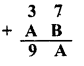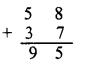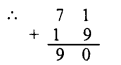# RD Sharma Class 8 Solutions Chapter 5 Playing With Numbers Ex 5.3

In this chapter, we provide RD Sharma Class 8 Solutions Chapter 5 Playing With Numbers Ex 5.3 for English medium students, Which will very helpful for every student in their exams. Students can download the latest RD Sharma Class 8 Solutions Chapter 5 Playing With Numbers Ex 5.3 Maths pdf, free RD Sharma Class 8 Solutions Chapter 5 Playing With Numbers Ex 5.3 Maths book pdf download. Now you will get step by step solution to each question.

### RD Sharma Solutions for Class 8 Chapter 5 Playing With Numbers Ex 5.3Download PDF

Solve each of the following cryptarithms.
Question 1.Solution:Values of A and B be from 0 to 9 In ten’s digit 3 + A = 9
∴ A = 6 or less.
∴ 7 + B = A = 6 or less
∴ 7 + 9 or 8 = 16 or 15
∴ But it is two digit number
B = 8
Then A = 5Question 2.Solution:
Values of A and B can be between 0 and 9
In tens digit, A + 3 = 9
∴ A = 9 – 3 = 6 or less than 6
In ones unit B + 7 = A = 6or less
∴ 7 + 9 or 8 = 16 or 15
But it is two digit number
∴ B = 8 and
∴ A = 5Question 3.Solution:Value of A and B can be between 0 and 9 In units place.
1+B = 0 ⇒1+B = 10
∴ B = 10 – 1 = 9
and in tens place
1 + A + 1 = B ⇒ A + 2 = 9
⇒ A = 9 – 2 = 7Question 4.Solution:
Values of A and.B can be between 0 and 9
In units place, B+1 = 8 ⇒ B = 8-1=7
In tens place A + B= 1 or A + B = 11
⇒ A + 7 = 11 ⇒ A =11-7 = 4

Question 5.Solution:Values of A and B can be between 0 and 9
In tens place, 2 + A = 0 or 2 + A=10
A = 10-2 = 8
In units place, A + B = 9
⇒ 8 + B = 9 ⇒ B = 9- 8 = 1Question 6.Solution:Values of A and B can be between 0 and 9
In hundreds place,Question 7.
Show that cryptarithm 4 x AB¯¯¯¯¯¯¯¯=CAB¯¯¯¯¯¯¯¯¯¯¯ does not have any solution.
Solution:It means that 4 x B is a numebr whose units digit is B
Clearly, there is no such digit
Hence the given cryptarithm has no solution.

All Chapter RD Sharma Solutions For Class 8 Maths

—————————————————————————–

All Subject NCERT Exemplar Problems Solutions For Class 8

All Subject NCERT Solutions For Class 8

*************************************************

I think you got complete solutions for this chapter. If You have any queries regarding this chapter, please comment on the below section our subject teacher will answer you. We tried our best to give complete solutions so you got good marks in your exam.

If these solutions have helped you, you can also share rdsharmasolutions.in to your friends.